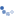# Material properties concrete & insulation materials,

Question:

Hi,

Could you please clarify the following:
To calculate the heat of hydration in our old software system we would just take the heat of hydration of the cement as shown on the technical data file of the cement (e.g. 260 kJ/kg) and multiply it with the cement content of 1m³ of concrete. This would give something like:

260 * 350 = 91000 kJ/m³ concrete.

How can I convert this correctly to the unit system used in Midas?

For the concrete:
We would like to use the following unit system:So what would be the values to use in the material properties? (specific heat & heat of hydration)And what about other materials, e.g. insulation materials (boundary conditions), do you maybe have some kind of material library with the values of different material as they should be used in Midas?